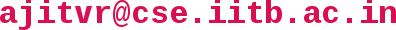## CS 215 - Data Interpretation and Analysis

Instructor: Ajit Rajwade and Suyash Awate
Office: SIA-218, KReSIT Building
Email:Lecture Venue:LH-101
Lecture Timings: Slot 8, Monday and Thursday 2:00 to 3:25 pm

Instructor Office Hours (in room SIA-218): Tuesdays 10:30 am to 11:30 am, Friday 11:00 am to 12 pm, or after class, or by appointment via email (also feel free to send queries over email)

Teaching Assistants: Ravi Mishra, Rajeev Kumar, Kalyani Dole, Pratik Kalshetti, Krishna Harsha, Siddhant Garg [Email ids: {ravimsr,rverma,kalyanid,pratikm,krishna.harsha,siddhant}@cse DOT ac DOT in ]

### Topics to be covered (tentative list)

• Descriptive statistics
• Discrete and continuous probability
• Random variables and expectation
• Special random variables: Gaussian, Bernoulli, Beta, Gamma, Uniform, Poisson, Exponential, Binomial, etc.
• Hypothesis testing
• Parameter estimation
• Regression
• Probability density estimation

### Intended Audience

2nd year BTech students from CSE

### Computational Resources

• Mid-sem exam: 25%
• Final exam (cumulative): 25%
• Programming and written assignments (about five): 35% - all to be done in groups of 2 students.
• Two pre-announced quizzes: 15% total

### Other Policies

• Attendance is mandatory. Students with less than 80% attendance may be given a DX grade.
• Assignments will be given out (typically) once every two or three weeks. They must be submitted on or before the deadline. No late assignments will be accepted. The programming components of the assignments will typically involve MATLAB, so you must be willing to learn it quickly.
• We will adopt a zero-tolerance policy against any forms of plagiarism or any other form of cheating. Just don't do it! In cases of plagiarism, givers and takers will both be considered equally responsible.
• This course is (inherently) cumulative. The syllabus for the final exam will include everything taught during the semester.

HW1, HW2

Quizzes

## Lecture Schedule:

### Content of the Lecture

18/07 (Mon)
• Introduction, course overview and course policies
• Descriptive statistics: key terminology
• Methods to represent data: frequency tables, bar/line graphs, frequency polygon, pie-chart
• Concept of frequency and relative frequency
• Cumulative frequency plots
• Interesting examples of histograms of intensity values in an image
21/07 (Thurs)
• Interesting examples of histograms of intensity values in an image
• Concept of mean, median, mode, percentile, standard deviation and variance with examples
• Mean as minimizer of total squared deviations, median as minimizer of sum of absolute deviations
• Chebyshev's inequality: two-sided and one-sided with examples
25/07 (Mon)
• Proof of Chebyshev's inequality: two-sided and one-sided
• Correlation coefficient: centered and uncentered versions, properties and examples
• Correlation and causation
• A demo of a simple MATLAB program
28/07 (Thurs)
• MATLAB demo.
• Please consult some of the MATLAB tutorials mentioned above on this webpage
• Examples covered in class: matrix and vector operations, code vectorization, functions for different types of plots and graphs, statistical functions (mean, median, variance, standard deviation)
01/08 (Mon)
• Discrete probability: sample space, event, composition of events: union, intersection, complement, exclusive or, De Morgan's laws
• Boole's and Bonferroni's inequalities
• Conditional probability, Bayes rule, False Positive Paradox
04/08 (Thurs)
• Random variable: concept, discrete and continuous random variables
• Probability mass function (pmf), cumulative distribution function (cdf) and probability density function (pdf)
• Expected value for discrete and continuous random variables
• Expected value of a function of a random variable
• The mean and the median as minimizers of squared and absolute losses respectively (with proofs)
• Variance and standard deviation, with alternate expressions
• Markov's and Chebyshev's inequality: with proofs
• Slides
• Read chapter 4 of the textbook
08/08 (Mon)
• Weak law of large numbers along with proof, statement of strong law of large numbers
• Gambler's fallacy
• Concept of joint PMF, PDF, CDF
• Concept of covariance, concept of mutual independence and pairwise independence
• Concept of moment generating function, two different proof of uniqueness of moment generating function for discrete random variables, properties of momenet generating functions
• Slides
• Read chapter 4 of the textbook
11/08 (Thurs)
• Concept of conditional PDF, CDF, PMF; conditional expectation and variance with examples
• Bernoulli, binomial and Poisson distributions and their properties: mean, variance, MGF, mode and median (in some cases)
18/08 (Thurs)
• Gaussian distribution: mean, variance, median, mode, MGF, other properties
• Central limit theorem: statement of theorem, MATLAB code to demo the theorem, and one application
22/08 (Mon)
• Proof of central limit theorem using the MGF
• de Moivre Laplace theorem - stated without proof
• Distribution of sample mean and sample covariance - chi-square distribution and its MGF for n degrees of freedom, genesis of the chi square distribution for n = 1
• Uniform distribution - mean, median, variance, MGF, application in sampling from arbitrary PMFs
25/08 (Thu)
• Exponential distribution: motivation, pdf, cdf, mean, variance, MGF, memorylessness
• Multinomial distribution: concept of mean vector and covariance matrix; mean, covariance and MGF of multinomial
• Introduction to hypergeometric distribution
29/08 (Mon)
• Concept of maximum likelihood estimation
• Maximum likelihood (ML) estimates for parameters of Bernoulli, Poisson, Gaussian and uniform distributions
• Concept of biased estimator and example (ML estimator of the variance of a Gaussian when the mean is also unknown)
• Introduction to the concept of the variance of an estimator
• Slides
• Read sections 7.1, 7.2, 7.7 of the textbook
1/09 (Thu)
• Bias, variance, mean squared error of an estimator, proof that mean squared error = squared bias + variance; consistency of an estimator
• Derivation of bias, MSE, variance for two different estimators of the parameter of a uniform distribution
• Concept of confidence interval - one-sided and two-sided, examples for mean of a Gaussian with known variance, variance of a Gaussian, mean of a Bernoulli (approximate)